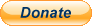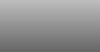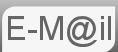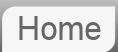# Hint to Riddle #8: Remainders

8. What is the smallest positive integer that leaves a remainder of 1 when divided by 2, remainder of 2 when divided by 3, a remainder of 3 when divided by 4, and so on up to a remainder of 9 when divided by 10?

Lets think about what numbers (N) would leave a remainder of 7 when divided by 8...

7, 15, 23, 31, 39, 47 and so on

It's pretty obvious at this stage that these numbers are all one less than the 8 times table. Which makes sense. If N were one higher it would be exactly divisible by 8. But it isn't so it leaves a remainder of 7.

What can we do with this observation? Well for a number N to leave a remainder of x-1 when divided by x we now know that N+1 must be a multiple of x. (x being 8 in the above example.)

In our question this must be true for x=2, 3, 4, ... ... 9, & 10

If you use this technique you may be able to arrive at an answer. But is it the lowest answer?

There is a tool here you can use to try different values of N

Note: no information is sent to me, the calculation is done entirely locally, on your computer.
Meaning N+1 is 2000

N+1 has the following factors:
2  3  4  5  6  7  8  9  10  All Factors

Where next?

© Nigel Coldwell 2004 -  – The questions on this site may be reproduced without further permission, I do not claim copyright over them. The answers are mine and may not be reproduced without my expressed prior consent. Please inquire using the link at the top of the page. Secure version of this page.PayPal
I always think it's arrogant to add a donate button, but it has been requested. If I help you get a job though, you could buy me a pint! - nigel